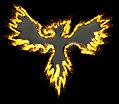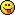Author Topic: X_SPRITE MidHandle?  (Read 3382 times)QubeX_SPRITE MidHandle?
« on: 2011-Jul-08 »
I'm currently doing a simple 3D particle system for my game and it would be very handy to be able to have the X_SPRITE handle to be centred rather than bottom middle as it is at the moment.

Is there any way to use / have X_SPRITE draw images with the x,y coors referring to the middle of the image rather than the bottom middle?Moru

• Prof. Inline
••• Posts: 1791Re: X_SPRITE MidHandle?
« Reply #1 on: 2011-Jul-08 »
No there is no easy way to do this at the moment. Also, don't try to play around with alpha if you are using x_sprite, it just doesn't work unless you sort the sprites so you draw the ones further away before you draw the close ones.

I would love a way to set the hotspot on both x_sprite and sprites in 2D so you can rotate around a certain spot, not just the middle. This was very handy in STOS basic on the Atari, can we have it in this nice modern thing too? :-)Kitty Hello

• code monkey
• Prof. Inline
••• Posts: 10817
• here on my island the sea says 'hello'Re: X_SPRITE MidHandle?
« Reply #2 on: 2011-Jul-08 »
not really. But it's just the "Y" position that you have to decrease. It's linear to the sprite size and the scaling of the sprite. I "think" the X_Sprite scaling of 1.0 is 100 pixels = 1.0 units in 3D.kanonet

• Prof. Inline
•• Posts: 1137Re: X_SPRITE MidHandle?
« Reply #3 on: 2011-Jul-08 »
Or you can create your own sprite commands, e.g. like this:

Code: (glbasic) [Select]
//! you just have to create one billboard and you can use it with different textures later
// \param num - objectnumber (using X_OBJ...), you need this to draw the billboard later
// \param sz - size of the billboard
FUNCTION CreateBillboard: num, sz
sz=sz/2
X_AUTONORMALS 1
X_OBJSTART num
X_OBJADDVERTEX  sz, -sz,  sz, 1, 1, RGB(255,255,255)
X_OBJADDVERTEX -sz, -sz,  sz, 0, 1, RGB(255,255,255)
X_OBJADDVERTEX  sz,  sz,  sz, 1, 0, RGB(255,255,255)
X_OBJADDVERTEX -sz,  sz,  sz, 0, 0, RGB(255,255,255)
X_OBJEND
ENDFUNCTION

//! draw a billboard (stretch and rotate it, if needed) - dont forget to texture it ;)
// \param num - objectnumber created with CreateBillboard()
// \param x,y,z - position (of the center) of the billboard
// \param cx,cy,cz - billboard will point at this (normaly this is the cameraposition)
// \param scale,rotate - make the billboard fit your needs ;)
FUNCTION DrawBillboard: num, x,y,z, cx,cy,cz, scale=1, rotation=0
X_MOVEMENT x, y, z
X_SCALING scale, scale, scale
X_ROTATION rotation, 0,0,1
z=cz-z
IF NOT z THEN INC z, 0.0000000000001
X_ROTATION -ATAN((cy-y)*COS(ATAN(cx-x, ABS(z))), ABS(z)), 1,0,0
X_ROTATION ATAN(cx-x, z), 0,1,0
X_DRAWOBJ num, 0
ENDFUNCTION
If you optimize this (use qqsin, create triangle instead of quad,...) and make it fit your needs (dont rotate it, if you dont need rotation,..), im sure it can be faster that X_SPRITE and it will do everythink you need.
Lenovo Thinkpad T430u: Intel i5-3317U, 8GB DDR3, NVidia GeForce 620M, Micron RealSSD C400 @Win7 x64Slydog

• Prof. Inline
••• Posts: 932
• KodeSourceRe: X_SPRITE MidHandle?
« Reply #4 on: 2011-Jul-08 »
kanoent, some great routines there!

I'm not experienced with matrices, but could billboarding be done faster / easier with a matrix?

A quick Google search gave me this: (OpenGL of course, in C)
Code: (glbasic) [Select]
glPushMatrix();
GLfloat matrix;
glGetFloatv(GL_MODELVIEW_MATRIX,matrix);
matrix = 1.0f;
matrix = 0.0f;
matrix = 0.0f;
matrix = 0.0f;
matrix = 1.0f;
matrix = 0.0f;
glPopMatrix();

http://nehe.gamedev.net/data/articles/article.asp?article=19
My current project (WIP) :: TwistedMaze <<  [Updated: 2015-11-25]Hemlos

• To boldy go where no pixel has gone before!
• Global Moderator
• Prof. Inline
••• Posts: 1621
• Particle HawkRe: X_SPRITE MidHandle?
« Reply #5 on: 2011-Jul-09 »
ive been playing with a 3d particle engine, i named spritez3d...
its not done yet, because of an issue with opengl..z ordering.

heres some stuff you can use...
you can make a particle out of anything...even missles...

This creates a centered billboard
Code: (glbasic) [Select]
FUNCTION EmitterSys_X_CreateSquare: Color
//flat square object, facing +z direction
LOCAL GenObjNum=GENX_OBJ()
X_AUTONORMALS 2 // smooth edges
X_OBJSTART GenObjNum
X_OBJNEWGROUP
X_OBJEND
RETURN GenObjNum
ENDFUNCTION

heres movement/rotation/scale code:

Code: (glbasic) [Select]
FUNCTION EmitterSys_X_Trans3d: x,y,z , Scale , rx,ry,rz // x,y,z, Scale, Rx,Ry,Rz //move,scale,rotate an object
// This function Handles 3d inputs for,
// Move, Scale, Rotate for 3d objects.

X_MOVEMENT x,y,z
X_SCALING Scale, Scale, Scale
IF rz<>0 THEN X_ROTATION rz,0,0,1
IF rx<>0 THEN X_ROTATION rx,1,0,0
IF ry<>0 THEN X_ROTATION ry,0,1,0

RETURN TRUE //pass completed, return=1 (BOOL=TRUE)
ENDFUNCTION

and heres the functions to properly rotate the particles to face the camera, or point a missle.

Code: (glbasic) [Select]
@FUNCTION X_Vec2Rot: vX , vY , vZ
//Vector XYZ is the input, transforms to rotations, for use with X_Rotation .
//Object Face must be created towards +Z, so a camera IN +Z pointing to 0,0,0 sees the face.
//Returns rotations X and Y
//
//
//
// Billboarding:
//   Call these before X_ROTATION:
//
// DistanceX = CameraX - BillBoardX
// DistanceY = CameraY - BillBoardY
// DistanceZ = CameraZ - BillBoardZ
// X_Vector2Rot( DistanceX , DistanceX , DistanceZ )
// X_ROTATION AngleX2,1,0,0
// X_ROTATION AngleY2,0,1,0
//
//
//
// Missles Guidance:
//   This will use the vector Vector(dist per frame) of the missle to guide the rotations.
//   Just use the real 3d Vector:
//
// X_Vector2Rotation( VectorX , VectorX , VectorZ )
// X_ROTATION AngleX2,1,0,0
// X_ROTATION AngleY2,0,1,0
//
// AngleX2 AngleY2 are your global memories for the x_rotation

vX2 = X_Distance( 0.0 , vY , 0.0 , vX , vY , vZ )
AngleX = ATAN(-vX2 , -vY ) + 90.0
AngleY = ATAN( -vX , -vZ ) + 180.0

ENDFUNCTION

@FUNCTION X_Distance: x0,y0,z0, x1,y1,z1
//This function is used by FaceObject() Operations.
//It gives a distance between two points in 3d space.
//Vector 1 = x0,y0,z0
//Vector 2 = x1,y1,z1
// The return is the true distance between these 2 points. (Hypotenuese)

LOCAL x , y , z , xx , yy , zz
x  = x0 - x1; y  = y0 - y1; z  = z0 - z1;
xx = x*x; yy = y*y; zz = z*z;
RETURN SQR( xx + yy + zz )

ENDFUNCTION

goodluck, i have been banging my head for years on this.
Volume_of_Earth(km^3) = 4/3*3.14*POW(6371.392896,3)QubeRe: X_SPRITE MidHandle?
« Reply #6 on: 2011-Jul-09 »
Thanks for the tipsI did think about using a plane instead of x_sprite but wondered if there was a quicker way to keep x_sprite centred at x,y,z coords. I'll have a play and see what happensQubeRe: X_SPRITE MidHandle?
« Reply #7 on: 2011-Jul-13 »
Yay!! sorted out my particles using x_sprite and getting past the z-ordering issueThe z-ordering was a pain and wasn't making sense. What works for me is to draw all your 3D objects first. Then the last thing you draw is your particles. However, in order to get past the z-ordering issue I have to do a custom sort which is :

Code: (glbasic) [Select]
// Particle[] is my array of type tParticle

ALPHAMODE 1
FOREACH blob in Particles[]
.... do your drawing of all particles - in my case X_SPRITE
NEXT

Code: (glbasic) [Select]
// tParticle is the type definition

FUNCTION z_sort:a AS tParticle, b AS tParticle
// CamX, Y, Z refers to the camera position
// Compare distance from camera particle to camera.

LOCAL temp1#, temp2#

temp1 = QSQR(((a.x - camX) * (a.x - camX)) + ((a.y - camY) * (a.y - camY)) + ((a.z - camZ) * (a.z - camZ)))
temp2 = QSQR(((b.x - camX) * (b.x - camX)) + ((b.y - camY) * (b.y - camY)) + ((b.z - camZ) * (b.z - camZ)))

IF temp1 > temp2 THEN RETURN -1
IF temp1 < temp2 THEN RETURN 1
ENDFUNCTION

Works spot on for me« Last Edit: 2011-Jul-13 by Qube »Hemlos

• To boldy go where no pixel has gone before!
• Global Moderator
• Prof. Inline
••• Posts: 1621
• Particle HawkRe: X_SPRITE MidHandle?
« Reply #8 on: 2011-Jul-14 »
Qube
nice work on the zorder function, PE's must use this in any case.

The problem im having with rendering is still visible due to opengl.
Maybe you know what i mean, when it looks like the particles run with a "choo choo train" visage as they move and die.
There is always one that overlaps all the other objects, no matter what, regardless of z order.
This is especially visible when firing hundreds of large overlapping particles moving on a path, that live and die in a second or two.
Maybe its just MY video cards?
Volume_of_Earth(km^3) = 4/3*3.14*POW(6371.392896,3)QubeRe: X_SPRITE MidHandle?
« Reply #9 on: 2011-Jul-15 »
I've noticed the outlines of particles when I use particles of vastly different sizes together. Luckily for what I need right now I'm OK with the above method as I only need a very basic particle system.

When my current game is finished though I *must* revisit this area as it's been driving me nuts and I *will* get it working properly